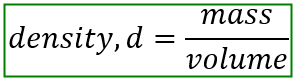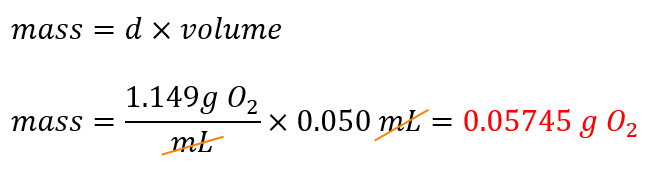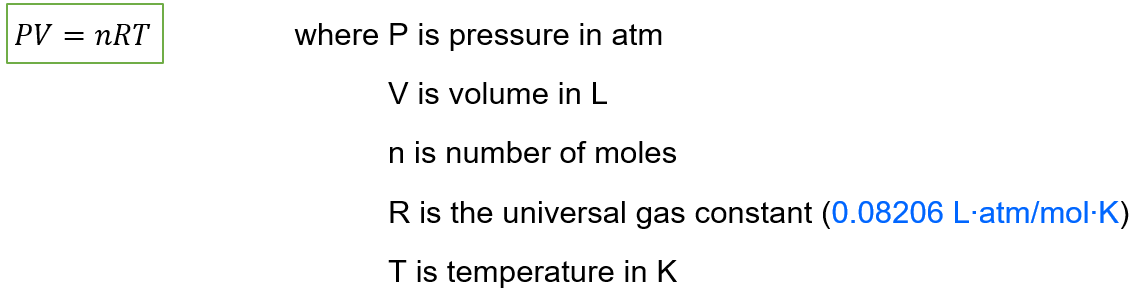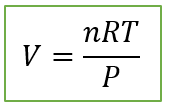# Problem: A person accidentally swallows a drop of liquid oxygen, O2(l), which has a density of 1.149 g/mL. Assuming the drop has a volume of 0.050 mL, what volume of gas will be produced in the person’s stomach at body temperature (37°C) and a pressure of 1.0 atm?

###### FREE Expert Solution

Density represents the mass of an object or compound within a given volume.Isolating mass:Assuming that the gas is ideal, the volume of O2 gas that will be produced can be obtained from the Ideal Gas Law.

The mathematical representation of the Ideal Gas Law is:Isolating volume:82% (206 ratings)###### Problem Details

A person accidentally swallows a drop of liquid oxygen, O2(l), which has a density of 1.149 g/mL. Assuming the drop has a volume of 0.050 mL, what volume of gas will be produced in the person’s stomach at body temperature (37°C) and a pressure of 1.0 atm?